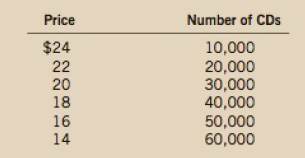Chapter 15, Problem 3PA

Chapter
Section
Textbook Problem

Johnny Rockabilly has just finished recording his latest CD. His record company’s marketing department determines that the demand for the CD is as follows:The company can produce the CD with no fixed cost and a variable cost of $5 per CD.a. Find total revenue for quantity equal to 10,000,20,000, and so on. What is the marginal revenue for each 10,000 increase in the quantity sold?b. What quantity of CDs would maximize profit? What would the price be? What would the profit be?c. If you were Johnny's agent, what recording fee would you advise johnny to demand from the record company? Why? Subpart (a): To determine Calculate total revenue and marginal revenue. Explanation Table – 1 shows the schedule of market demand for the CD. Table – 1  Price Quantity 24 10,000 22 20,000 20 30,000 18 40,000 16 50,000 14 60,000 Total revenue is calculated using the following formula: Total Revenue=Price×Quantity (1) Substitute the respective values in Equation (1) to calculate the total revenue at quantity of 10,000 units. Total Revenue=Price×Quantity=24×10,000=240,000 Thus, total revenue is$240,000.

Marginal Revenue is calculated using the following formula:

Marginal Revenue=Total RevenuecurrentTotal RevenuePreviousQuantitycurrentQuantityprevious (2)

Substitute the respective values in Equation (2) to calculate the marginal revenue at the output level of 20,000 units

Subpart (b):

To determine
Total cost and marginal cost.

Subpart (c):

To determine
Recommended quantity.

Still sussing out bartleby?

Check out a sample textbook solution.

See a sample solution

The Solution to Your Study Problems

Bartleby provides explanations to thousands of textbook problems written by our experts, many with advanced degrees!

Get Started

Find more solutions based on key concepts